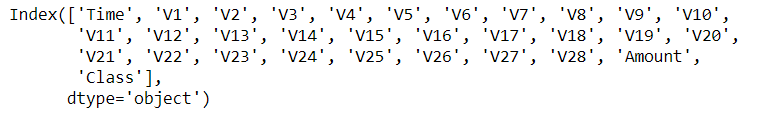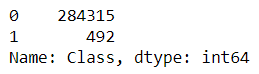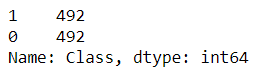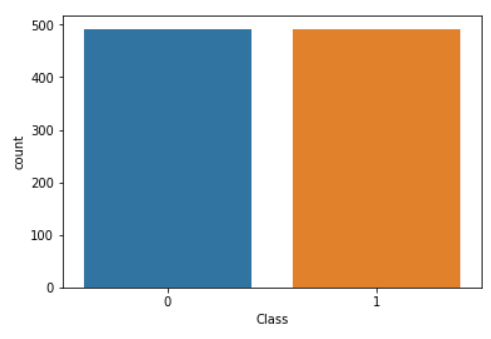Prerequisite: What are Skewed or Imbalanced datasets?

Random Undersampling in Data Science is about creating a balanced dataset by removing some of the data which belongs to the highly dominated class.

Let us take an example to understand this. We will be working with the Credit Card dataset.

Importing the libraries

Code

```import numpy as np
import pandas as pd
import matplotlib.pyplot as plt
import seaborn as sns
```

Importing the dataset and creating a data frame

Code

```data = pd.read_csv(”creditcard.csv”)
```

Let us check what columns are there in the dataset

Code

```data.columns
```

OutputCode

```data[‘Class’].value_counts()
```

OutputHere “Class” is a categorical variable, with values 0 and 1. Where 0 means “No Fraud” and 1 means “Fraud”. We can clearly see that the “No Fraud” data dominates over “Fraud” data with a very high percentage. Hence this dataset is highly skewed and cannot be further used for training the model, otherwise, it will overfit the dominating class.

To make it usable for training purposes, we need to first balance the dataset, so that each class in the dataset is in equal proportion with the other class. To do this, we can use the Random Undersampling method.

### Random Undersampling

We will first shuffle the entire dataset.

Code

```data = data.sample(frac=1)
```

As we can see from above that there were only 492 samples of “Fraud” data. So we need to extract out 492 samples of the “No Fraud” from the dataset.

Code

```fraud_data = data.loc[data[‘Class’] == 1]
non_fraud_data = data.loc[data[‘Class’] == 0][:492]
```

Because now we have equal samples of Fraud and Non-Fraud data, the skewness in the dataset has gone. Thus the new data we have is normally distributed.

Let us concatenate both the datasets — fraud_data & non_fraud_data

Code

```normal_distributed_data = pd.concat([fraud_data, non_fraud_data])
```

Because we simply concatenated both the datasets one after the other, we need to shuffle the newly merged data.

Code

```new_data = normal_distributed_data.sample(frac=1, random_state=42)
```

So we have finally performed, Random Undersampling. Let us now examine our new dataset.

Code

```new_data[‘Class’].value_counts()
```

OutputSo, both the classes have 492 values each. Which means that the dataset is now equally distributed.

#### Visualization of the new dataset

Code

```sns.countplot(‘Class’, data=new_data)
```

Output